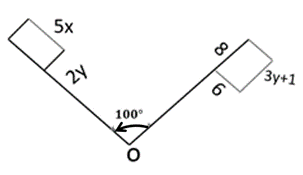Mathematics
Easy

Question

# The quadrilateral is rotated about O. What is the value of x?## 2    3    4    5Hint:

## The correct answer is: 2

### Step 1 : Rotation is isometry.finding y2y = 6y = 3Step 2 :  with help of y calculate x5x = 3y + 1 (substitute y = 3)Step 3 :  solving for x5x = 3(3) + 15x = 10x = 2so , x= 2 is the right answer .

Compare corresponding sides as they are equal .

### Related Questions to study#### With Turito Foundation.#### Get an Expert Advice From Turito.